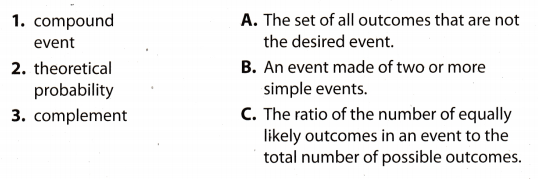# Texas Go Math Grade 7 Module 6 Answer Key Theoretical Probability and Simulations

Refer to our Texas Go Math Grade 7 Answer Key Pdf to score good marks in the exams. Test yourself by practicing the problems from Texas Go Math Grade 7 Module 6 Answer Key Theoretical Probability and Simulations.

## Texas Go Math Grade 7 Module 6 Answer Key Theoretical Probability and Simulations

Write each fraction as a decimal and a percent.

Question 1.
$$\frac{3}{4}$$ ____________
Divide 3 by 4. Write a decimal point and insert extra zeros in the dividend.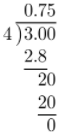The result is 0.75.
Move the decimal point two places to the right and add the “%” sign.
0.75 = 75%
$$\frac{3}{4}$$ = 75%

Question 2.
$$\frac{2}{5}$$ ____________
Divide 2 by 5. Write a decimal point and insert extra zeros in the dividend.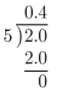The result is 0.40.
Move the decimal point two places to the right and add the “%” sign.
0.40 = 40%
$$\frac{2}{5}$$ = 40%

Question 3.
$$\frac{9}{10}$$ ____________
Divide 9 by 10. Write a decimal point and insert extra zeros in the dividend.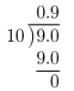The result is 0.90.
Move the decimal point two places to the right and add the “%” sign.
0.90 = 90%
$$\frac{9}{10}$$ = 90%

Question 4.
$$\frac{7}{20}$$ ____________
Divide 7 by 20. Write a decimal point and insert extra zeros in the dividend.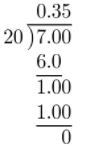The result is 0.35.
Move the decimal point two places to the right and add the “%” sign.
0.35 = 35%
$$\frac{7}{20}$$ = 35%

Question 5.
$$\frac{7}{8}$$ ____________
Divide 7 by 8. Write a decimal point and insert extra zeros in the dividend.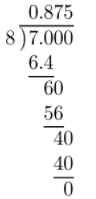The result is 0.875.
Move the decimal point two places to the right and add the “%” sign.
0.875 = 87.5%
$$\frac{7}{8}$$ = 87.5%

Question 6.
$$\frac{1}{20}$$ ____________
Divide 1 by 20. Write a decimal point and insert extra zeros in the dividend.The result is 0.05.
Move the decimal point two places to the right and add the “%” sign.
0.05 = 5%
$$\frac{1}{20}$$ = 5%

Question 7.
$$\frac{19}{25}$$ ____________
Divide 19 by 25. Write a decimal point and insert extra zeros in the dividend.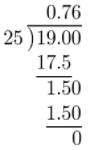The result is 0.76.
Move the decimal point two places to the right and add the “%” sign.
0.76 = 76%
$$\frac{19}{25}$$ = 76%

Question 8.
$$\frac{23}{50}$$ ____________
Divide 23 by 50. Write a decimal point and insert extra zeros in the dividend.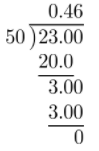The result is 0.46.
Move the decimal point two places to the right and add the “%” sign.
0.46 = 46%
$$\frac{23}{50}$$ = 46%

Find each difference.

Question 9.
1 – $$\frac{1}{5}$$ ___________
1 – $$\frac{1}{5}$$ = $$\frac{5}{5}$$ – $$\frac{1}{5}$$ Write 1 as $$\frac{5}{5}$$.
= $$\frac{5-1}{5}$$ Subtract the numerators.
= $$\frac{4}{5}$$

Question 10.
1 – $$\frac{2}{9}$$ ___________
1 – $$\frac{2}{9}$$ = $$\frac{9}{9}$$ – $$\frac{2}{9}$$ Write 1 as $$\frac{9}{9}$$.
= $$\frac{9-2}{9}$$ Subtract the numerators.
= $$\frac{7}{9}$$

Question 11.
1 – $$\frac{8}{13}$$ ___________
1 – $$\frac{8}{13}$$ = $$\frac{13}{13}$$ – $$\frac{8}{13}$$ Write 1 as $$\frac{13}{13}$$.
= $$\frac{13-8}{13}$$ Subtract the numerators.
= $$\frac{5}{13}$$

Question 12.
1 – $$\frac{3}{20}$$ ___________
1 – $$\frac{3}{20}$$ = $$\frac{20}{20}$$ – $$\frac{3}{20}$$ Write 1 as $$\frac{20}{20}$$.
= $$\frac{20-3}{20}$$ Subtract the numerators.
= $$\frac{17}{20}$$

Multiply. Write each product in simplest form.

Question 13.
$$\frac{8}{15}$$ × $$\frac{5}{8}$$ ___________
$$\frac{8}{15}$$ × $$\frac{5}{8}$$ = $$\frac{8}{15}$$ × $$\frac{5}{8}$$ Divide numerators and denominators by the common factors.
= $$\frac{1}{3}$$ × $$\frac{1}{1}$$
= $$\frac{1 \cdot 1}{3 \cdot 1}$$
= $$\frac{1}{3}$$

Question 14.
$$\frac{2}{9}$$ × $$\frac{3}{4}$$ ___________
$$\frac{2}{9}$$ × $$\frac{3}{4}$$ = $$\frac{2}{9}$$ × $$\frac{3}{4}$$ Divide numerators and denominators by the common factors.
= $$\frac{1}{3}$$ × $$\frac{1}{2}$$
= $$\frac{1 \cdot 1}{3 \cdot 2}$$
= $$\frac{1}{6}$$

Question 15.
$$\frac{9}{16}$$ × $$\frac{12}{13}$$ ___________
$$\frac{9}{16}$$ × $$\frac{12}{13}$$ = $$\frac{9}{16}$$ × $$\frac{12}{13}$$ Divide numerators and denominators by the common factors.
= $$\frac{9}{4}$$ × $$\frac{3}{13}$$
= $$\frac{9 \cdot 3}{4 \cdot 13}$$
= $$\frac{27}{52}$$

Question 16.
$$\frac{7}{10}$$ × $$\frac{5}{28}$$ ___________
$$\frac{7}{10}$$ × $$\frac{5}{28}$$ = $$\frac{7}{10}$$ × $$\frac{5}{28}$$ Divide numerators and denominators by the common factors.
= $$\frac{1}{2}$$ × $$\frac{1}{4}$$
= $$\frac{1 \cdot 1}{2 \cdot 4}$$
= $$\frac{1}{8}$$

Visualize Vocabulary

Use the ✓ words to complete the graphic.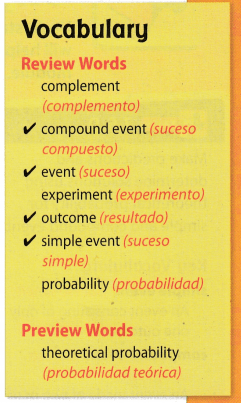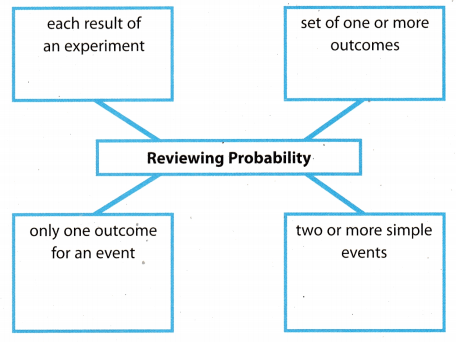Understand Vocabulary

Match the term on the left to the correct expression on the right.